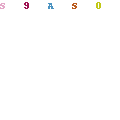﻿ Real Numbers: Exercise 1.4

# CHAPTER 1 : Real Numbers

## Exercise 1.4

1. Without actually performing the long division, state whether the following rational numbers will have a terminating decimal expansion or a non-terminating repeating decimal expansion.
1.2.3.4.5.6.7.8.9.10.2. Write down the decimal expansions of those rational numbers in Question 1 above which have terminating decimal expansions.
1.2.3.4.5.6.7.8.9.10.3. The following real numbers have decimal expansions as given below. In each case, decide whether they are rational or not. If they are rational, and of the form, what can you say about the prime factors of q?
1.2.3.